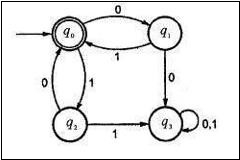# Construct RE for given finite automata with Arden’s theorem

There are two methods for converting a Deterministic Finite Automata (DFA) to Regular expression (RE). These methods are as follows −

• Arden's Theorem Method.
• State Elimination Method.

Let us understand the Arden's Theorem method in detail.

## Arden's Theorem

Let P and Q be the two regular expressions.

If P does not contain null string, then the following equation in R, viz R = Q + RP,

Which has a unique solution by R = QP*

Here,

• The finite automata (FA) does not have epsilon moves.
• It must have only initial state q1.
• Its states are q1, q2, q3,....qn. The final state may be some qi where i<=n.
• qi is the regular expression representing a set of strings accepted by the finite automata even though qi is a final state.

Now let us consider a problem, that how to construct given finite automata with the help of Arden's theorem.

## Problem

Find out the regular expression for the given finite automata by applying Arden's theorem.## Solution

Let us see the equations.

q0 = q1 + q20+ E

q1 =q00

q2 =q01

q3 = q10 + q21+ q3(0+1)

Let us solve q0 first, as shown below−

q0 = q1 + q20+ E

q0 = q1 + q010+ E

q0 = q0(01 +10)+ E

q0 = E(01+10)*

q0 = (01+10)*

·: R = Q+ RP

=> QP* where

R =q0 ,Q =e, P= (01 + 10)

Thus, the regular expression will be as follows −

r = {01+10)*

Since q0 is a final state, we are interested in q0 only.